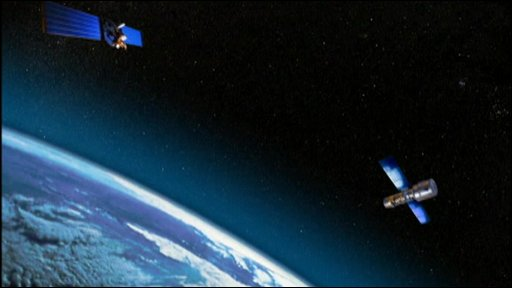Classical Mechanics

# Deriving Kepler's LawsTwo satellites each of mass $$m_1 = 3.00 \text{ kg}$$ and mass $$m_2 = 6.00 \text{ kg}$$ revolve around the same planet. The respective radii of their orbits are $$r_1 = 2.00 \times 10^5 \text{ m}$$ and $$r_2 = 1.80 \times 10^6 \text{ m}.$$ If the time period of the satellite of mass $$m_1$$ is $$T_1 = 4.00 \times 10^5 \text{ s},$$ what is that of the satellite of mass $$m_2?$$

Assumptions and Details

• The universal gravitational constant is $$G=6.67 \times 10^{-11} \text{ N}\cdot\text{m}^2\text{/kg}^2.$$

A box of mass $$m = 3 \text{ kg}$$ is placed on the edge of a merry-go-round of radius $$r= 4 \text{ m}.$$ The coefficient of static friction between the box and the merry-go-round is $$\mu = 0.3 .$$ What is the merry-go-round's speed squared at the moment the box slides off?

Assumptions and Details

• The gravitational acceleration is $$g= 10 \text{ m/s}^2.$$

A satellite of mass $$m = 2.00 \times 10^3 \text{ kg}$$ revolves around a planet of mass $$M = 6.00 \times 10^{16} \text{ kg},$$ with constant speed. If the radius of the orbit is $$r = 2.00 \times 10^5 \text{ m},$$ what is the satellite's approximate speed?

Assumptions and Details

• The universal gravitational constant is $$G=6.67 \times 10^{-11} \text{ N}\cdot\text{m}^2\text{/kg}^2.$$

A satellite of mass $$m = 5.00 \times 10^3 \text{ kg}$$ revolves around a planet of mass $$M = 5.00 \times 10^{16} \text{ kg},$$ at constant speed. If the radius of the orbit is $$r = 3.00 \times 10^5 \text{ m} ,$$ what is the approximate period of the revolution?

Assumptions and Details

• The universal gravitational constant is $$G=6.67 \times 10^{-11} \text{ N}\cdot\text{m}^2\text{/kg}^2.$$

Two identical satellites each of mass $$m = 6.00 \times 10 ^3 \text{ kg}$$ revolve around a planet of mass $$M = 5.00 \times 10^{16} \text{ kg}.$$ If the respective radii of their orbits are $$r_1 = 2.00 \times 10^5 \text{ m}$$ and $$r_2 = 1.80 \times 10^6 \text{ m} ,$$ what is the ratio between the speeds of the two satellites?

Assumptions and Details

• The universal gravitational constant is $$G=6.67 \times 10^{-11} \text{ N}\cdot\text{m}^2\text{/kg}^2.$$
×

Problem Loading...

Note Loading...

Set Loading...